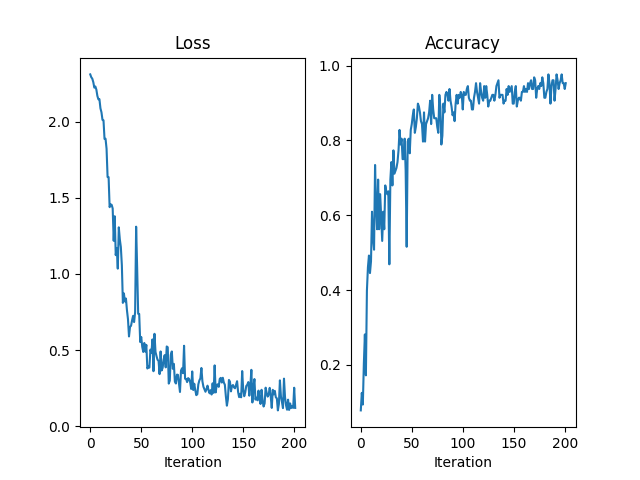# Diagonal Gauss-Newton Second order optimizer

A simple second-order optimizer with BackPACK on the classic MNIST example from PyTorch. The optimizer we implement uses uses the diagonal of the GGN/Fisher matrix as a preconditioner, with a constant damping parameter;

$x_{t+1} = x_t - \gamma (G(x_t) + \lambda I)^{-1} g(x_t),$

where

$\begin{split}\begin{array}{ll} x_t: & \text{parameters of the model} \\ g(x_t): & \text{gradient} \\ G(x_t): & \text{diagonal of the Gauss-Newton/Fisher matrix at x_t} \\ \lambda: & \text{damping parameter} \\ \gamma: & \text{step-size} \\ \end{array}\end{split}$

Let’s get the imports, configuration and some helper functions out of the way first.

import matplotlib.pyplot as plt
import torch

from backpack import backpack, extend
from backpack.extensions import DiagGGNMC

BATCH_SIZE = 128
STEP_SIZE = 0.05
DAMPING = 1.0
MAX_ITER = 200
PRINT_EVERY = 50
DEVICE = torch.device("cuda:0" if torch.cuda.is_available() else "cpu")
torch.manual_seed(0)

model = torch.nn.Sequential(
torch.nn.Conv2d(1, 20, 5, 1),
torch.nn.ReLU(),
torch.nn.MaxPool2d(2, 2),
torch.nn.Conv2d(20, 50, 5, 1),
torch.nn.ReLU(),
torch.nn.MaxPool2d(2, 2),
torch.nn.Flatten(),
torch.nn.Linear(4 * 4 * 50, 500),
torch.nn.ReLU(),
torch.nn.Linear(500, 10),
).to(DEVICE)

loss_function = torch.nn.CrossEntropyLoss().to(DEVICE)

def get_accuracy(output, targets):
"""Helper function to print the accuracy"""
predictions = output.argmax(dim=1, keepdim=True).view_as(targets)
return predictions.eq(targets).float().mean().item()


## Writing the optimizer

To compute the update, we will need access to the diagonal of the Gauss-Newton, which will be provided by Backpack in the diag_ggn_mc field, in addition to the grad field created py PyTorch. We can use it to compute the update direction

$(G(x_t) + \lambda I)^{-1} g(x_t)$

for a parameter p as

$\texttt{p.grad / (p.diag_ggn_mc + damping)}$
class DiagGGNOptimizer(torch.optim.Optimizer):
def __init__(self, parameters, step_size, damping):
super().__init__(parameters, dict(step_size=step_size, damping=damping))

def step(self):
for group in self.param_groups:
for p in group["params"]:
step_direction = p.grad / (p.diag_ggn_mc + group["damping"])


## Running and plotting

After extend-ing the model and the loss function and creating the optimizer, the only difference with a standard PyTorch training loop will be the activation of the DiagGGNMC extension using a with backpack(DiagGGNMC()): block, so that BackPACK stores the diagonal of the GGN in the diag_ggn_mc field during the backward pass.

extend(model)
extend(loss_function)
optimizer = DiagGGNOptimizer(model.parameters(), step_size=STEP_SIZE, damping=DAMPING)

losses = []
accuracies = []
for batch_idx, (x, y) in enumerate(mnist_loader):

x, y = x.to(DEVICE), y.to(DEVICE)

outputs = model(x)
loss = loss_function(outputs, y)

with backpack(DiagGGNMC()):
loss.backward()

optimizer.step()

# Logging
losses.append(loss.detach().item())
accuracies.append(get_accuracy(outputs, y))

if (batch_idx % PRINT_EVERY) == 0:
print(
"Iteration %3.d/%3.d " % (batch_idx, MAX_ITER)
+ "Minibatch Loss %.3f  " % losses[-1]
+ "Accuracy %.3f" % accuracies[-1]
)

if MAX_ITER is not None and batch_idx > MAX_ITER:
break

fig = plt.figure()

axes.plot(losses)
axes.set_title("Loss")
axes.set_xlabel("Iteration")

axes.plot(accuracies)
axes.set_title("Accuracy")
axes.set_xlabel("Iteration")Iteration   0/200 Minibatch Loss 2.313  Accuracy 0.078
Iteration  50/200 Minibatch Loss 0.581  Accuracy 0.844
Iteration 100/200 Minibatch Loss 0.359  Accuracy 0.883
Iteration 150/200 Minibatch Loss 0.239  Accuracy 0.891
Iteration 200/200 Minibatch Loss 0.251  Accuracy 0.938

Text(0.5, 23.52222222222222, 'Iteration')
`

Total running time of the script: ( 0 minutes 30.434 seconds)

Gallery generated by Sphinx-Gallery